#### 3.1.5Geometrical Interpretation of FEM

The manner in which the FEM was presented in this work makes the geometrical interpretation of the method a natural consequence. In this section it will be shown that this concept is intimately connected to the error of the linear space discretization of the FEM. In order to proceed smoothly through the upcoming discussion, consider that the linear space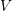is endowed with inner products for real functions defined by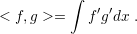(3.19)

Furthermore, consider the norm induced by the inner product (3.19) as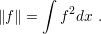(3.20)

Consequently, the variational form (3.5) and the discrete variational form (3.10) can be rewritten as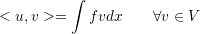(3.21)

and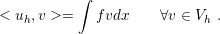(3.22)

The discrete problem solution (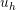) is computed by FEM as seen in the previous sections, but the exact solution of the BVP problem is given by solving the continuous variational form. Therefore, it is natural to ask how distantis from. The answer of this question leads to a geometrical view of the FEM.

Consider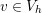. The functionalso belongs tosince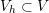. Therefore, for every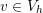(3.22) can be subtracted from (3.21), given by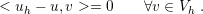(3.23)

As an inner product, (3.19) must hold the orthogonality property (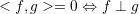). This means that the discretization error (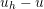) is orthogonal to the space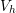. As a consequence, the solution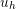is the orthogonal projection of the exact solutionin. Fig. 3.6 illustrates this principle.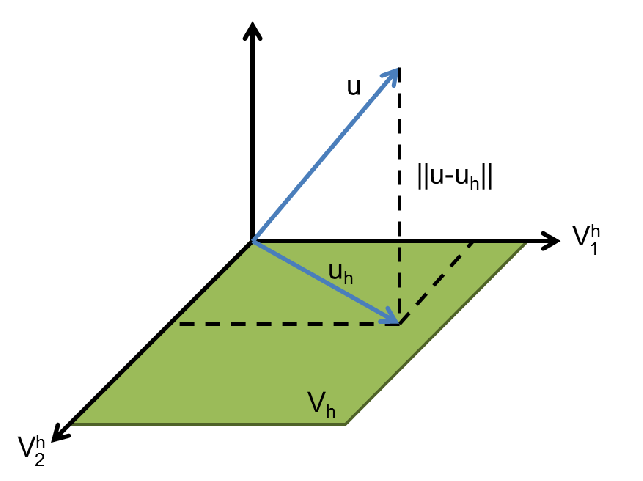Figure 3.6.: Geometrical interpretation of Galerkin’s method. The solution () of the original problem is projected () in the space defined by the basis of. Galerkin’s method can be understood as the procedure to find this projection.

As a result of the orthogonal projection, the elementis the closest function toin comparison to all elements of. Hence, the error of discretization is bounded according to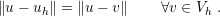(3.24)

The relation (3.24) will not be proved here, but it is intuitively clear when orthogonal projections are kept in mind. In conclusion, FEM provides the best approximation of the exact solutionin the discretized space, when the norm (3.20) is considered.

At this point, the advantage of the original Galerkin’s imposition for the solutionand the test functionbecomes evident –and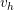, respectively, for the discrete formulation – to reside in the same linear space. The formulation gains some formal support, especially regarding the discretization error, since it is guaranteed that the FEM solution is the best choice in a particular space.